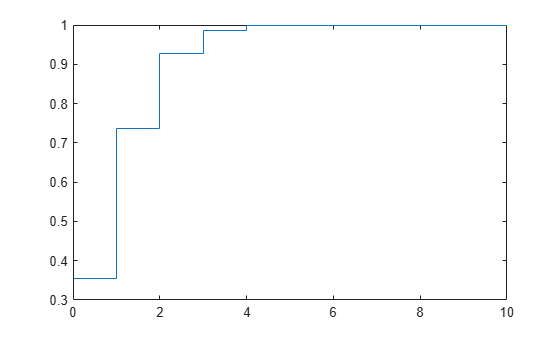## Hypergeometric Distribution

### Definition

The hypergeometric pdf is

`$y=f\left(x|M,K,n\right)=\frac{\left(\begin{array}{c}K\\ x\end{array}\right)\left(\begin{array}{c}M-K\\ n-x\end{array}\right)}{\left(\begin{array}{c}M\\ n\end{array}\right)}$`

### Background

The hypergeometric distribution models the total number of successes in a fixed-size sample drawn without replacement from a finite population.

The distribution is discrete, existing only for nonnegative integers less than the number of samples or the number of possible successes, whichever is greater. The hypergeometric distribution differs from the binomial only in that the population is finite and the sampling from the population is without replacement.

The hypergeometric distribution has three parameters that have direct physical interpretations.

• M is the size of the population.

• K is the number of items with the desired characteristic in the population.

• n is the number of samples drawn.

Sampling “without replacement” means that once a particular sample is chosen, it is removed from the relevant population for all subsequent selections.

### Examples

#### Compute and Plot Hypergeometric Distribution CDF

This example shows how to compute and plot the cdf of a hypergeometric distribution.

Compute the cdf of a hypergeometric distribution that draws 20 samples from a group of 1000 items, when the group contains 50 items of the desired type.

```x = 0:10; y = hygecdf(x,1000,50,20);```

Plot the cdf.

`stairs(x,y)`The x-axis of the plot shows the number of items drawn that are of the desired type. The y-axis shows the corresponding cdf values.